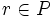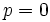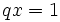# PID implies one-dimensional

This article gives the statement and possibly, proof, of an implication relation between two integral domain properties. That is, it states that every integral domain satisfying the first integral domain property (i.e., principal ideal domain) must also satisfy the second integral domain property (i.e., one-dimensional domain)
View all integral domain property implications | View all integral domain property non-implications |Get help on looking up integral domain property implications/non-implications
Get more facts about principal ideal domain|Get more facts about one-dimensional domain

## Statement

A principal ideal domain is a one-dimensional domain. In other words, in a principal ideal domain, every nonzero prime ideal is a maximal ideal.

## Facts used

1. every proper ideal is contained in a maximal ideal

## Proof

Given: A principal ideal domain$R$, a nonzero prime ideal$P$ of$R$.

To prove:$P$ is a maximal ideal of$R$.

Proof: Suppose$P$ is not maximal in$R$. Then, by fact (1), there exists a maximal ideal$Q$ of$R$ properly containing$P$. Since$R$ is a principal ideal domain, there exist$q \in Q, p \in P$ such that$(q) = Q, (p) = P$. In particular, we can write$p = qr$ for some$r \in R$.

Note that since$P$ is prime, and$q \notin P$ because$P$ is properly contained in$Q$, we have$r \in P$. Thus,$r = px$ for some$x \in R$. This gives$p = p(qx)$, giving$p(1 - qx) = 0$. Since$R$ is an integral domain, this forces either$p = 0$ (a contradiction to$P$ being nonzero) or$qx = 1$ (a contradiction to$Q$ being a proper ideal). Thus, we have the required contradiction.